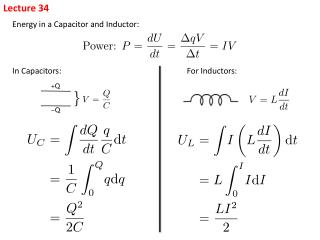DownloadDownload PresentationLecture 34

# Lecture 34

Download Presentation## Lecture 34

- - - - - - - - - - - - - - - - - - - - - - - - - - - E N D - - - - - - - - - - - - - - - - - - - - - - - - - - -
##### Presentation Transcript

1. Lecture 34 Energy in a Capacitor and Inductor: In Capacitors: For Inductors:

2. Mechanical Analogy: Mass Spring System Corresponds to: LC circuits oscillate much in the same way a mass spring system oscillates

3. LC Circuit Basic Equations: Loop Equation: Power Equation: Conservation of Energy:

4. Solving the Loop Equation – Analogy to Mass-Spring System Solution: Comment: General Solution: (δ is the initial phase angle of the oscillation) Often set δ= 0, where at t = 0, the initial condition is chosen to be x = xmax

5. LC Circuit Analogy

6. Check: where

7. RLC Circuit – Loop Equation Graph of Q and I of Dampened Oscillation: Each term can be positive or negative. PL + PC stays negative Always positive